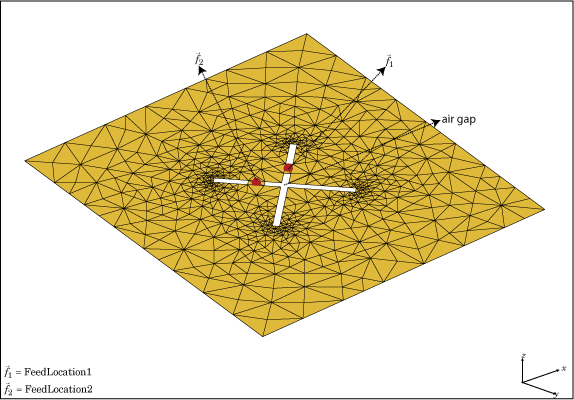# customArrayMesh

Create 2-D custom mesh antenna array on X-Y plane

## Description

The `customArrayMesh` object creates an array represented by a 2-D custom mesh on the X-Y plane. You can provide an arbitrary array mesh to the Antenna Toolbox™ and analyze this mesh as a custom array for port and field characteristics.## Creation

### Description

example

``` `customarray = customArrayMesh(points,triangles,numfeeds)` creates a 2-D array represented by a custom mesh, based on the specified points and triangles.```

### Input Arguments

expand all

Points in custom mesh, specified as a `2-by-N` or `3-by-N` matrix of Cartesian coordinates in meters. N is the number of points. In case you specify a `3-by-N` integer matrix, the Z-coordinate must be zero or a constant value. This value sets the `'Points'` property in the custom array mesh.

Example: `load planarmesh.mat`; ```c = customArrayMesh(p,t,4)```. Creates a custom array mesh from the points, `p`, extracted from the `planarmesh.mat` file.

Data Types: `double`

Triangles in the mesh, specified as a `4-by-M` matrix. M is the number of triangles. The first three rows are indices to the points matrix and represent the vertices of each triangle. The fourth row is a domain number useful for identifying separate parts of an array. This value sets the `'Triangles'` property in the custom array mesh.

Example: `load planarmesh.mat`; ```c = customArrayMesh(p,t,4)```. Creates a custom array mesh from the triangles, `t`, extracted from the `planarmesh.mat` file.

Data Types: `double`

Number of feeding points in array, specified as a scalar. By default, the number of feed points are 2.

Example: `load planarmesh.mat`; ```c = customArrayMesh(p,t,4)```. Creates a custom array mesh requiring `4` feed points.

Data Types: `double`

## Properties

expand all

Points in a custom mesh, specified as a `2-by-N` or `3-by-N` matrix of Cartesian coordinates in meters. N is the number of points.

Data Types: `double`

Triangles in the mesh, specified as a `4-by-M` matrix. M is the number of triangles.

Data Types: `double`

Number of feeding points in the array, specified as a scalar.

Data Types: `double`

Feed locations of array, specified as Cartesian coordinates in meters. Feed location is a read-only property. To create a feed for the 2–D custom mesh, use the `createFeed` method.

Data Types: `double`

Excitation amplitude of antenna elements, specified as a scalar or a non-negative vector. Set the property value to `0` to model dead elements.

Example: `'AmplitudeTaper'`,`3`

Data Types: `double`

Phase shift for antenna elements, specified as a scalar or a real vector in degrees.

Example: `'PhaseShift'`,[`3 3 0 0`]. Creates a custom array mesh of four antennas with phase shifts specified.

Data Types: `double`

## Object Functions

 `show` Display antenna, array structures or shapes `info` Display information about antenna or array `createFeed` Create feed locations for custom array `beamwidth` Beamwidth of antenna `charge` Charge distribution on antenna or array surface `correlation` Correlation coefficient between two antennas in array `current` Current distribution on antenna or array surface `EHfields` Electric and magnetic fields of antennas; Embedded electric and magnetic fields of antenna element in arrays `impedance` Input impedance of antenna; scan impedance of array `mesh` Mesh properties of metal, dielectric antenna, or array structure `pattern` Radiation pattern and phase of antenna or array; Embedded pattern of antenna element in array `patternAzimuth` Azimuth pattern of antenna or array `patternElevation` Elevation pattern of antenna or array `returnLoss` Return loss of antenna; scan return loss of array `sparameters` Calculate S-parameter for antenna and antenna array objects

## Examples

collapse all

Load a custom mesh and create an array.

```load planarmesh.mat; c = customArrayMesh(p,t,2);```

Create feeds for the custom array mesh.

`createFeed(c,[0.07,0.01],[0.05,0.05], [-0.07,0.01],[-0.05,0.05])`

Calculate the impedance of the array.

`Z = impedance(c,1e9)`
```Z = 1×2 complex 64.3925 - 7.8288i 58.9598 -11.3558i ```

 Balanis, C.A. Antenna Theory: Analysis and Design. 3rd Ed. New York: Wiley, 2005.